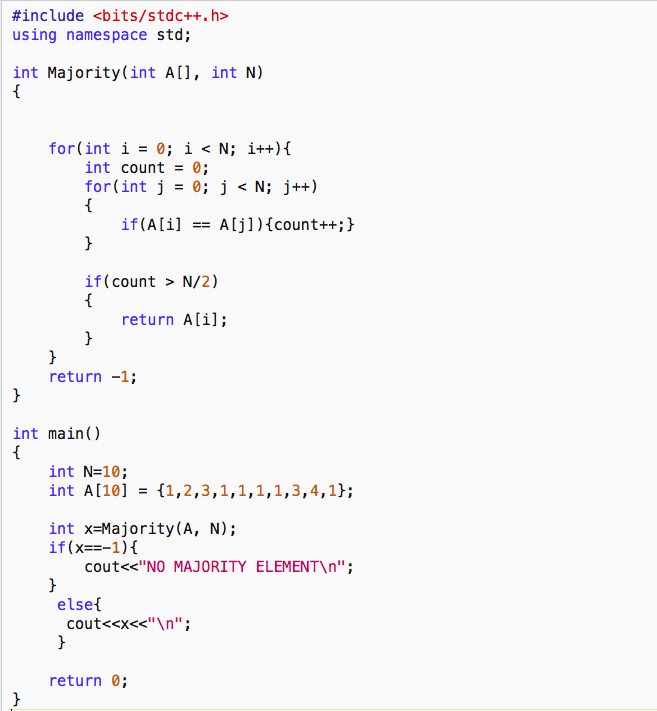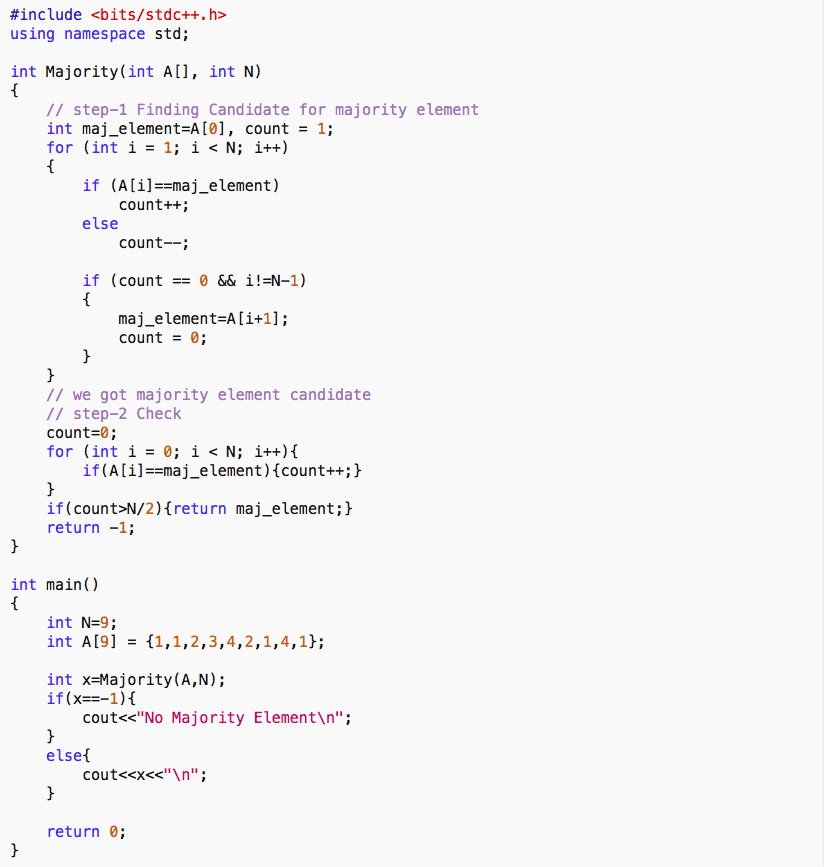## Moore’s Voting Algorithm

Aim- Finding Majority Element in an efficient way. Here majority element refers to an element that occurs more than N/2 times in an array of size N.

Ex. {1,1,3,4,5,1,1,1} has 1 as majority element as 1 occur more than 4(N/2 where N=8) times.

## Brute Force Approach

- The brute force solution is to use two nested ‘for’ loops and count for the occurrence of each value and if that element occurs more than N/2 times simply return it.Analysis-

1. Time Complexity- As we are using two nested for loops time complexity is O(n^2)

2. Space Complexity- Auxiliary space by our function Majority is O(1).

## A More Efficient Approach-

Idea- So basically we can maintain a hash map of all values present in an array with their frequencies. And then we can simply loop over these values to find the majority element.

Time Complexity -O(n)

Space Complexity- O(n)

## Highly Efficient Approach(Moore’s Voting)-

Approach- Point to emphasize is there can be at max 1 majority element. Our algorithm has two steps first we will find the potential candidate for the majority element than in step-2 we will verify this as there can be cases where there is no majority element.

Step-1 : Concept is if we subtract the occurrence of the majority element with the total occurrence of all other elements we will be left with at least 1 occurrence of the majority element.

Ex- {1,1,3,4,5,1,1}

Occurrence of 1 - 4

Other occurrence- 3

Subtracting we get +1

Whereas if we see the same approach for any other number we will get non-positive results.

---> So what we can do is in a loop, assume 1st element as the majority element with count=1 and move forward if the same number is encountered increase count by 1.If at any point count turns out to be 0. Then just take the next number as the majority element and put count as 1 and follow the same approach. After iterating on the full array the element obtained is a candidate for majority element.

Step-2 : Again we iterate over all elements in the array and count the total occurrence of the element found in step-1 if this number turns out to be greater than N/2 then this is our majority element else there is no majority element in the array.Analysis-

1. Time Complexity - We are iterating over the whole array two times hence Time complexity is O(n) .

2. Space Complexity - Auxiliary space used is O(1).

Practice-

Once you are comfortable with this there is a good problem on this concept slightly tricky where you need to find a number with occurrence more than N/3 in O(n) time and O(1) space.

Hint - You need to apply Moore’s voting Algorithm twice with appropriate changes.